Courses

# Theory & Procedure, Diode Characteristics Class 12 Notes | EduRev

## JEE : Theory & Procedure, Diode Characteristics Class 12 Notes | EduRev

The document Theory & Procedure, Diode Characteristics Class 12 Notes | EduRev is a part of the JEE Course Physics Class 12.
All you need of JEE at this link: JEE

Objective

To draw the I-V characteristic curve of a p-n junction diode in forward bias and reverse bias.

Theory

Semiconductors

Semiconductors are materials with electrical conductivity intermediate between that of a conductor and an insulator. In semiconducting materials thermal energy is enough to cause a number of electrons to release from valance band to conduction band, in which they are relatively free. Common semiconducting materials are silicon, germanium, gallium, arsenide etc.

Semiconductors are classified as;

Intrinsic semiconductors :Pure semiconducting materials like crystalline form of germanium and silicon, with equal concentration of electrons and holes.

Extrinsic semiconductors :Semiconducting material with the addition of suitable impurity atoms through doping.

Extrinsic semiconductors can be p-type or n-type depending on the impurities added to it. A p- type semiconductor is formed when adding pentavalent impurities like phosphorus, arsenic, antimony etc. to an intrinsic semiconductor. If the impurities added are trivalent atoms, we get the n- type semiconductor.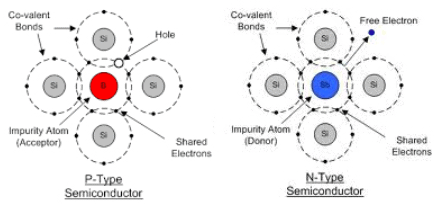Semiconductor Diodes

Semiconductor diode is simply the combination of a p-type and an n-type material. It is formed by doping half of the silicon crystal with trivalent impurity (p-type) and the other half with pentavalent impurity (n-type). It has the characteristics of passing current in one direction only. If there is no voltage is applied across the junction, electrons will diffuse through the junction to p - side and holes will diffuse through the junction to n - side and they combine with each other. Thus the acceptor atom near the p - side and donor atom near n – side are left unutilized and is called the depletion layer. An electron field is generated by these uncovered charges which called the barrier potential. This opposes further diffusion of carriers and is known as depletion region.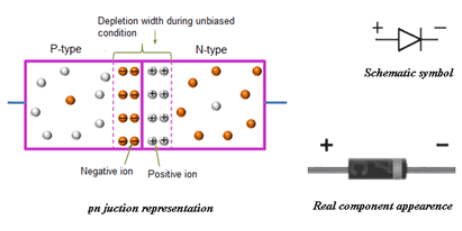Biasing in diodes

Biasing in general means the application of dc voltage across the terminals of a device for a particular operation. Two types of biasing are possible in a p-n junction diode. They are;

Forward Biasing :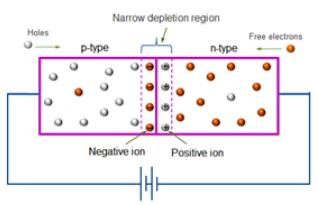Forward biasing occurs when the positive end of the diode is connected to the positive terminal of the battery, and its negative end to the negative terminal of the battery. Here, majority carriers from both sides move towards and cross the junction and current flows through the junction. This current is known as the forward current and is the order of 10-3 A. The size of the depletion layer decreases in forward biasing.

Reverse Biasing :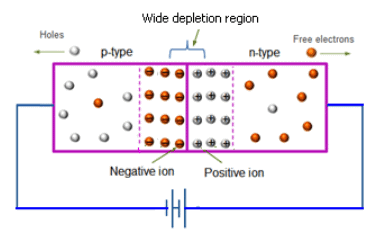Reverse biasing occurs when the positive end of the diode is connected to the negative terminal of the battery, and its negative end to the positive terminal of the battery. Here, majority carriers from both sides move away from the junction and thus no current flows through the junction. A very small current will made at the junction due to the movement of minority charge carriers across the junction.

Characteristics of a p-n junction diode

It generally shows the relation between bias voltage and current of a diode. The V-I characteristics of a diode can be forward or reverse. The graph showing the forward bias voltage and forward current is known as the forward characteristics, and that showing the reverse bias voltage and reverse current is known as the reverse characteristics.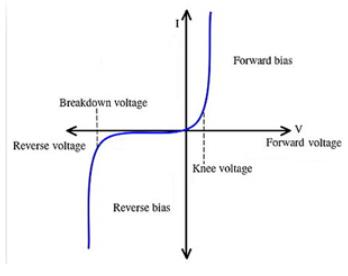The forward characteristics of a diode is non linear. The forward current increases slowly in the beginning and shows a sudden rise at a certain value of forward voltage. This voltage is known as the threshold voltage or Knee voltage. This is because the resistance is very low in forward biased condition. The current in the reverse bias is due to the flow of minority carriers. The reverse current shows a sudden increase at a particular region. The corresponding voltage is termed as the reverse breakdown voltage.

Learning Outcomes

• Students understand p-n junction diodes and their working.
• Students learn the different types of biasing in diodes.
• Students understand the concept of diode characteristics.
• Students learn the different terms associated with p-n junction diodes.

Materials Required

• A p-n junction diode
• 3 V battery
• 50 V battery
• High resistance rheostat
• 0-3 V voltmeter
• 0-50 V voltmeter
• 0-100 mA ammeter
• 0-100 µA ammeter
• One way key
• Connecting wires

Real Lab Procedure

Forward V-I Characteristics :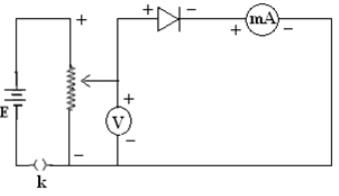• Connections are made as per the connection diagram.
• Insert the key. Arrange the sliding contact of the rheostat to minimum.
• Now, gently move the rheostat contact to provide a positive bias voltage.
• Note the voltmeter and milli ammeter readings.
• Repeat the process by increasing the forward current in equal steps by changing the rheostat slider.
• It can be noted that, initially the current increase very slowly. For a certain value of voltage, it shows a sharp increase. The corresponding voltage represents the knee voltage of that diode.
• Plot a graph with forward voltage along X axis and forward current along Y axis. The graph shows the forward V-I characteristics of the given p-n junction diode.

Reverse V-I Characteristics :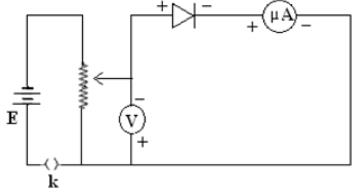• Make the circuit diagram as shown in the figure.
• Insert the key. Arrange the sliding contact of the rheostat to maximum.
• Move the sliding contact of the rheostat to provide a reverse bias voltage.Note the voltmeter and micro         ammeter readings.
• Note the voltmeter and micro ammeter readings.
• Repeat the process by changing the reverse voltage in equal steps.
• The current increases slowly in the beginning and then rapidly when the reverse voltage becomes a certain value. This voltage is known as the reverse breakdown voltage.
• Plot a graph with reverse voltage along X axis and reverse current along Y axis. The graph shows the reverse V-I characteristics of the given p-n junction diode.

Simulator Procedure (as performed through the Online Labs)

• Select the characteristics of diode from the drop down list.
• Select the diode from the drop down list.
• To see the circuit diagram, click on the 'Show circuit diagram' check box seen inside the simulator window.
• Connections can be made as seen in the circuit diagram by clicking and dragging the mouse from one connecting terminal to the other connecting terminal of the devices to be connected.
• Drag the key to insert it.
• You can change the rheostat resistance using the slider.
• You can select the room temperature using the slider.
• You can notice the corresponding ammeter and voltmeter readings.
• To redo the experiment, click on the ‘Reset’ button.

Observations

Forward V-I Characteristics:

 Sl. No. Forward bias voltage, Vf(V) Forward current, If(mA) 1 2 3 4 5 6 7 8 9 10

Reverse V-I Characteristics:

 Sl. No. Reverse bias voltage, Vr (V) Reverse current, Ir  (µA) 1 2 3 4 5 6 7 8 9 10

Calculations

For the forward characteristics of the given p-n junction diode, a graph is plotted with forward voltage along X axis and forward current along Y axis. The forward current shows a sudden increase at certain forward voltage, which is known as the knee voltage.

For the reverse characteristics of the given p-n junction diode, a graph is plotted with reverse voltage along X axis and reverse current along Y axis. I t is noted that at a certain reverse voltage, the reverse current reaches its maximum level. Further increase in voltage does not increase this current. It is the reverse saturation current. However, with further increase in reverse voltage, the current shows a rapid rise at a certain value. It is known as the reverse breakdown voltage.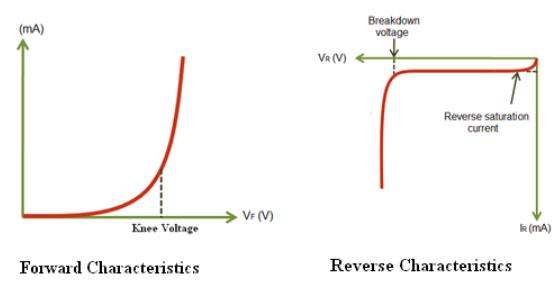Result

The forward and reverse characteristics of the given p-n junction diode is drawn.

Offer running on EduRev: Apply code STAYHOME200 to get INR 200 off on our premium plan EduRev Infinity!

## Physics Class 12

132 videos|259 docs|130 tests

,

,

,

,

,

,

,

,

,

,

,

,

,

,

,

,

,

,

,

,

,

,

,

,

;